# Year 5 Maths Printable Worksheets

Our year 5 maths worksheets are tailored to the Y5 maths curriculum and cover all the core maths topics including angles, percentages, mental maths, shapes and much more. Whether it's finding the difference between the area and perimeter of geometric shapes or adding and subtracting fractions, you will be sure to find a year 5 maths worksheet to suit your child's needs.

Filters

### Converting decimals to fractions

Students convert 1 or 2 digit decimals to fractions.

Age: 9 - 10
1
2
3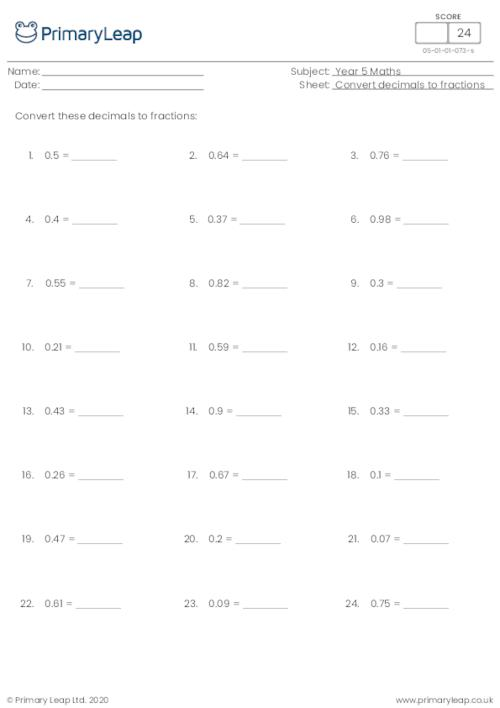### Converting fractions to decimals (denominator of 10 or 100)

Students practise converting fractions to decimals with a denominator of 10 or 100.

Age: 9 - 10
1
2
3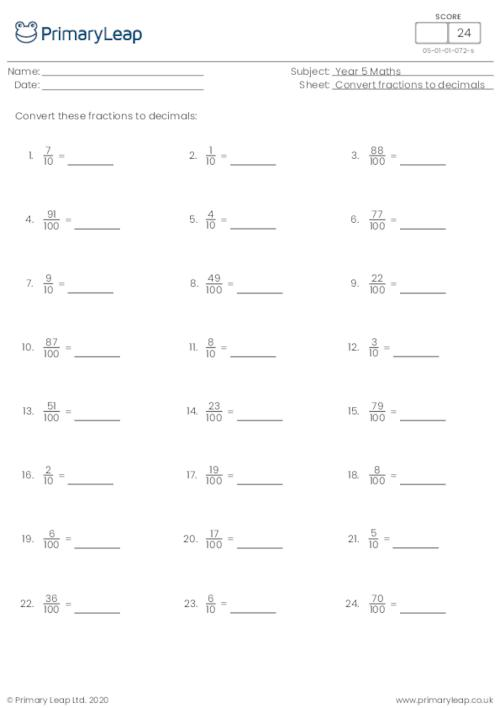### Converting fractions to decimals (denominator of 100)

Students practise converting fractions to decimals with a denominator of 100.

Age: 9 - 10
1
2
3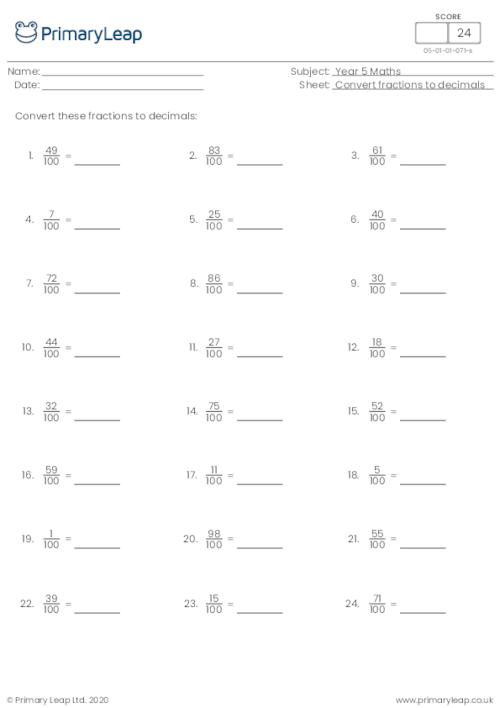### Subtracting fractions (same denominator)

This maths worksheet asks students to find the difference by subtracting the fractions.

Age: 9 - 10
1
2
3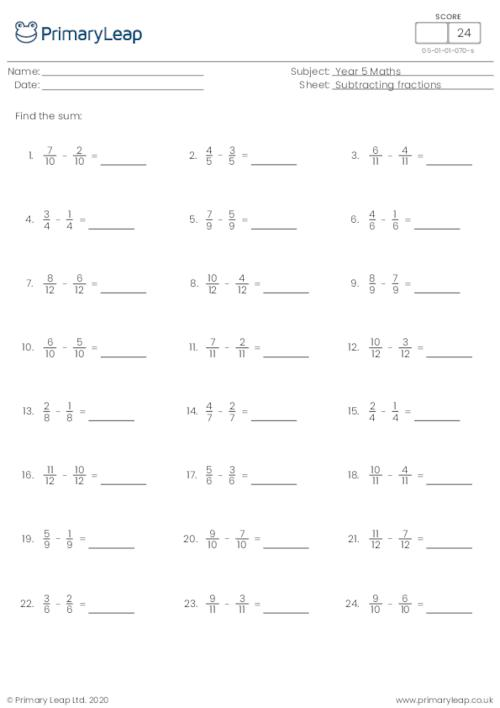This maths worksheet asks students to find the sum by adding the fractions.

Age: 9 - 10
1
2
3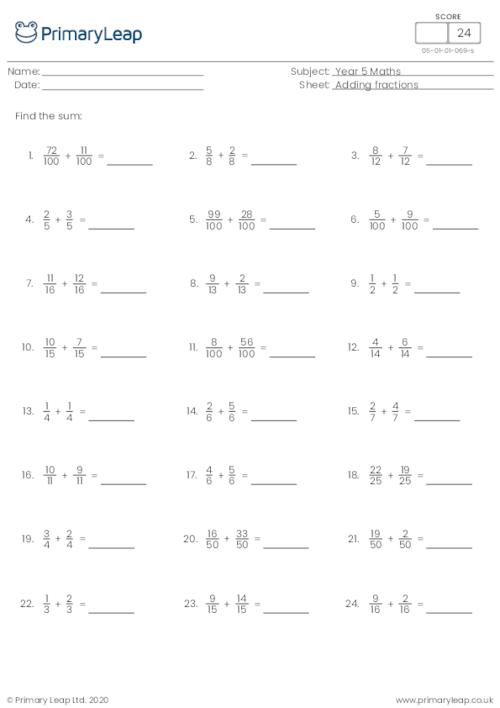### Recognising years written in Roman numerals

Children read the Roman numerals and write the years in numbers. They are then given 5 high grossing films and the year that they were released. They write the year using Roman numerals.

Age: 9 - 10
1
2
3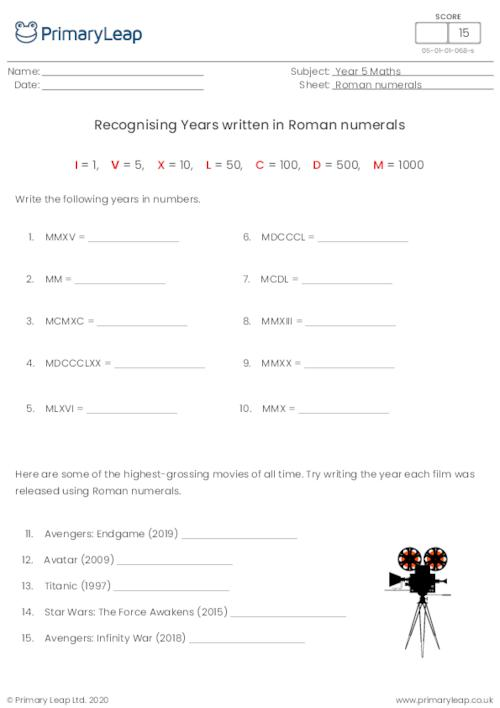### Writing Roman Numerals to 1000

Children write the numbers up to 1000 as Roman numerals.

Age: 9 - 10
1
2
3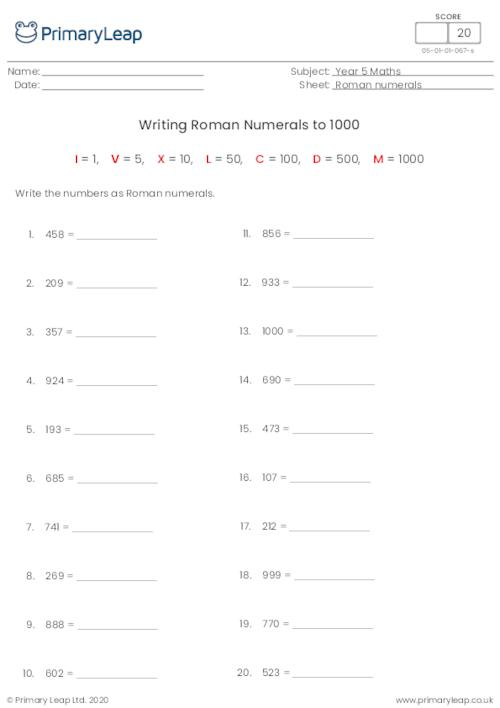### Reading Roman Numerals to 1000

Children read the Roman numerals up to 1000 and write the number in normal form.

Age: 9 - 10
1
2
3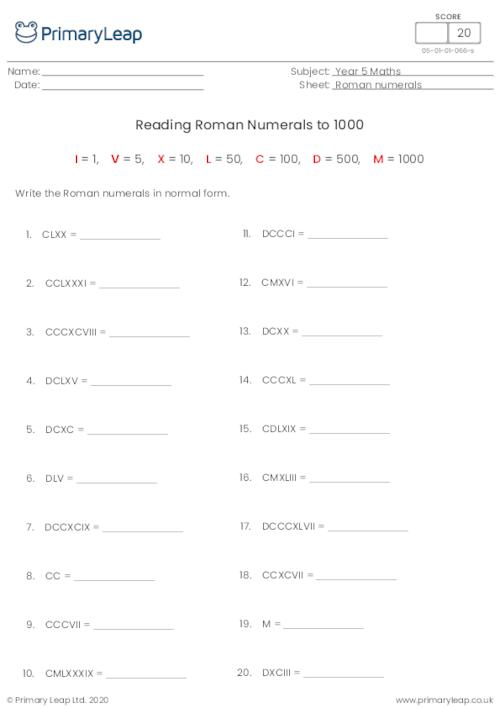### Roman Numerals Chart to 2000

This Roman numerals chart showing numbers up to 2000 can be used as an aid to help children complete maths activities on Roman numerals.

Age: 9 - 10
1
2
3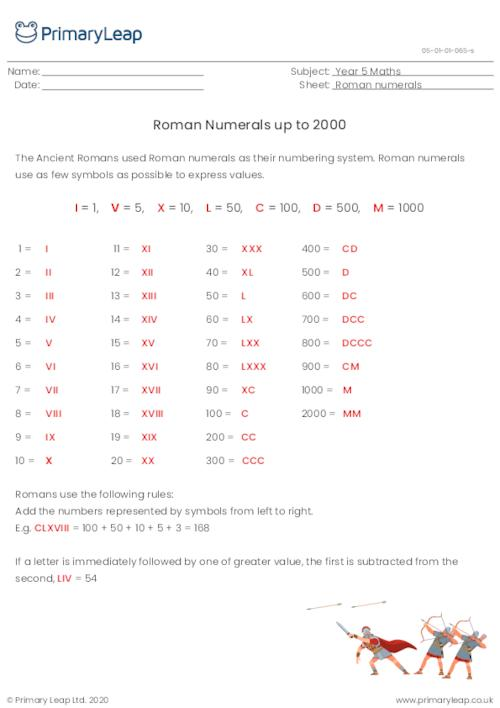Students are asked to complete the addition sums with 1 digit decimals.

Age: 9 - 10
1
2
3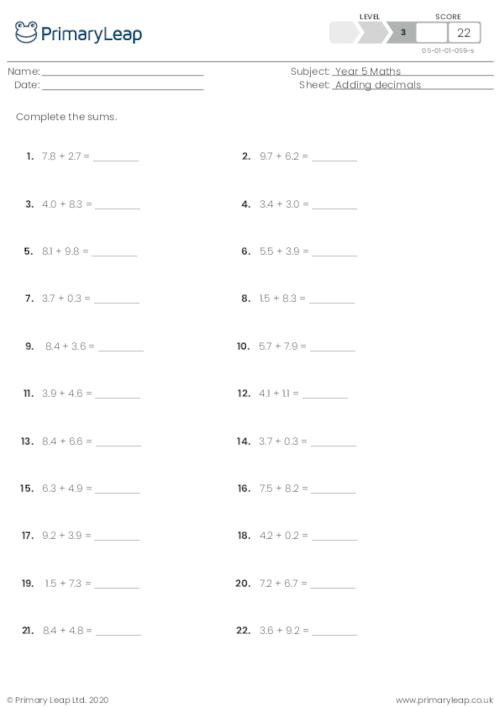Age: 9 - 10
1
2
3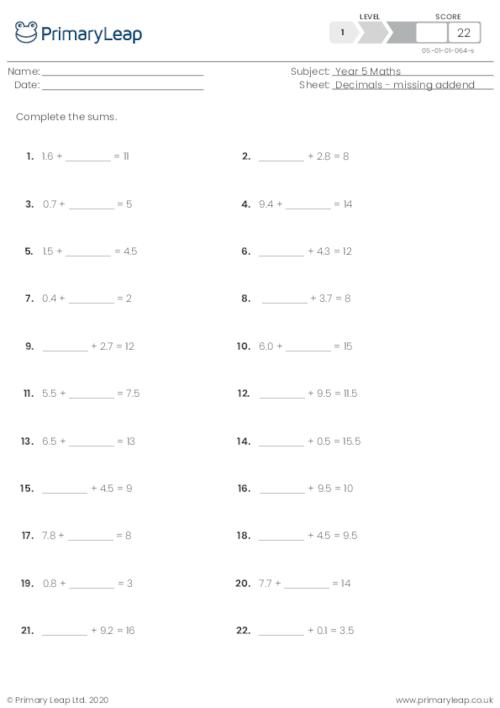Age: 9 - 10
1
2
3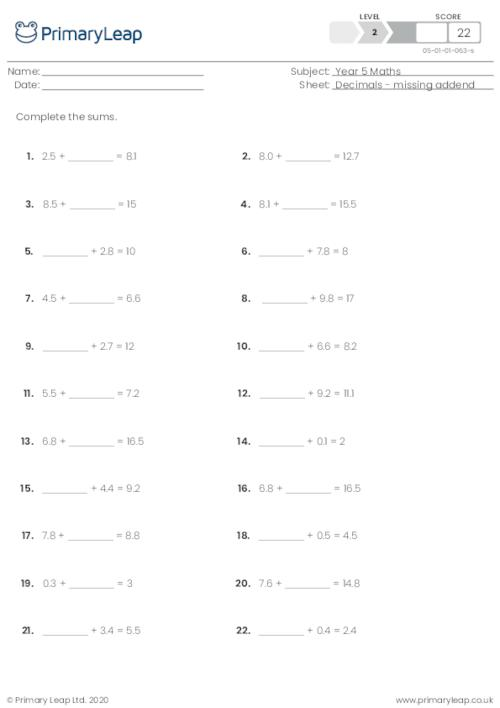Age: 9 - 10
1
2
3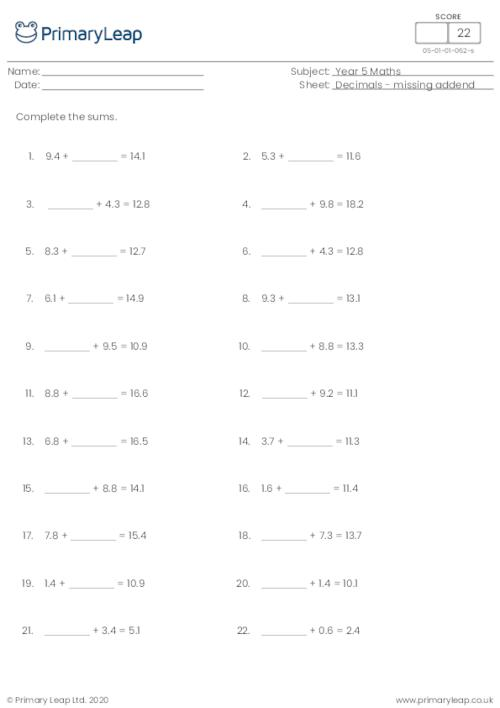This maths resource includes 22 addition sums with 1 digit decimals.

Age: 9 - 10
1
2
3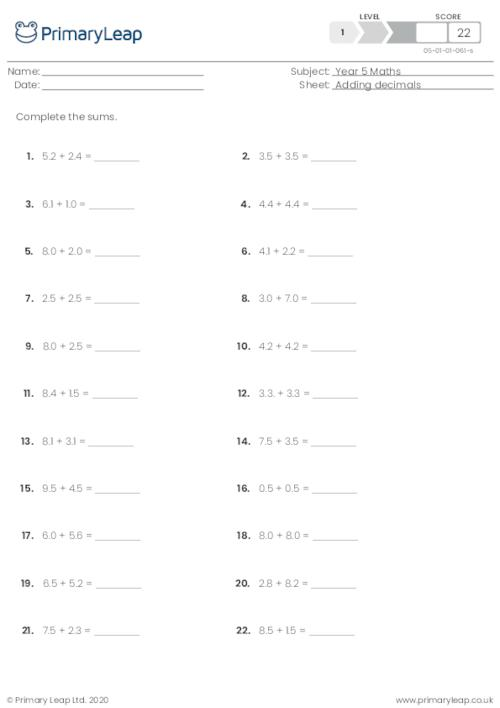Students are asked to complete the addition sums with 1 digit decimals.

Age: 9 - 10
1
2
3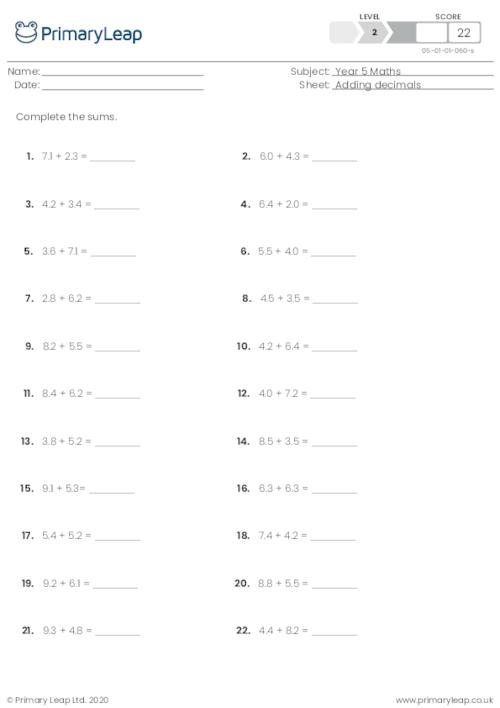### Balancing Equations - Addition and subtraction

Maths: Students are asked to work out the sums. This resource will help with addition and subtraction.

Age: 9 - 10
1
2
3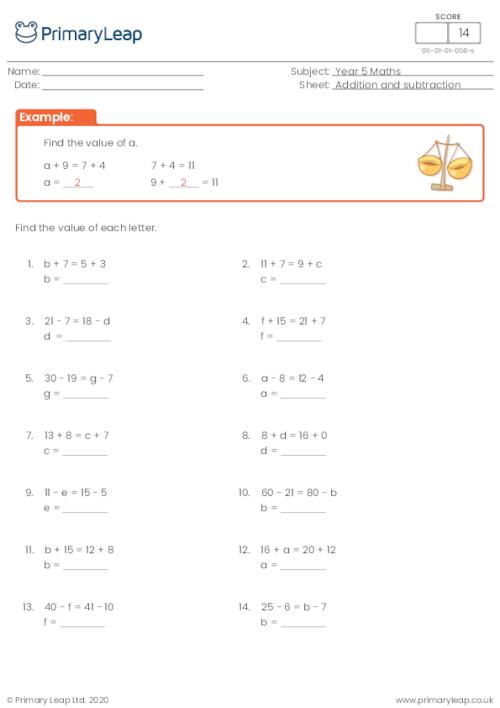### Balancing Equations - Multiplication and division

Maths: Students are asked to work out the sums. This resource will help with multiplication and division.

Age: 9 - 10
1
2
3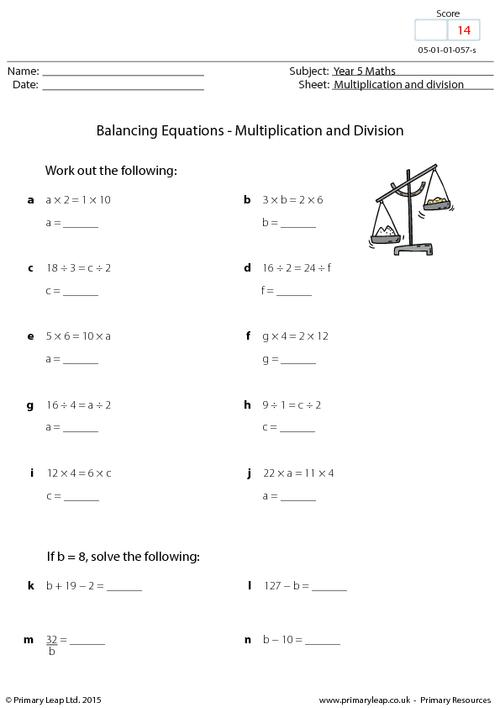### Division: 5 digits by 1 - set 5

Math: Division - 21 division questions dividing 5 digits by 1.

Age: 9 - 10
1
2
3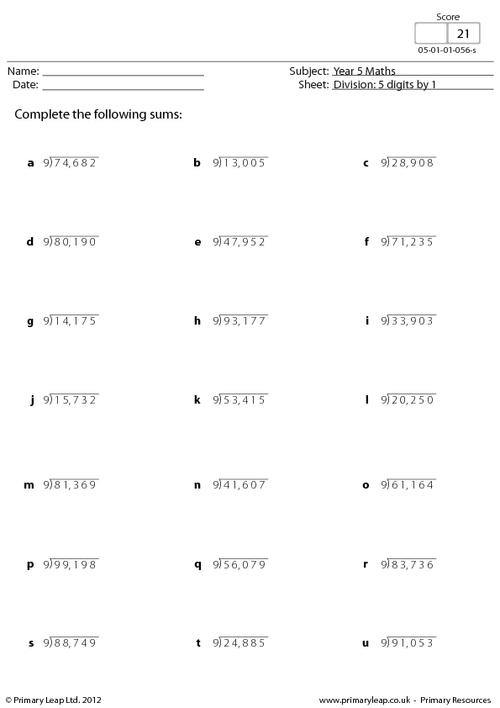### Division: 5 digits by 1 - set 4

Math: Division - 21 division questions dividing 5 digits by 1.

Age: 9 - 10
1
2
3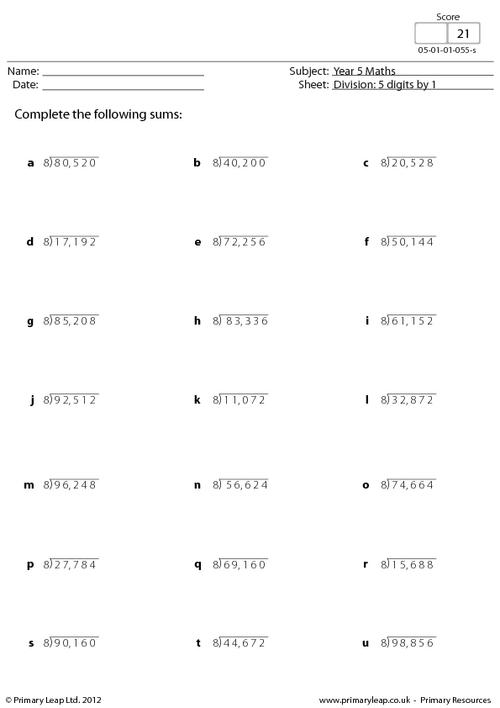### Division: 5 digits by 1 - set 3

Math: Division - 21 division questions dividing 5 digits by 1.

Age: 9 - 10
1
2
3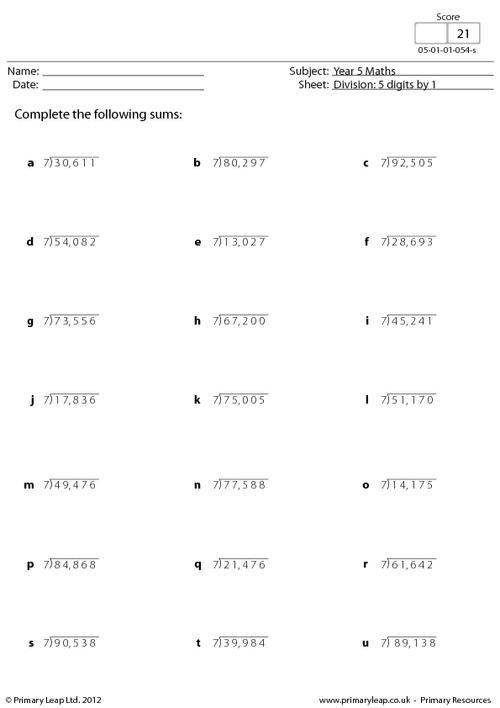### Division: 5 digits by 1 - set 2

Math: Division - 21 division questions dividing 5 digits by 1.

Age: 9 - 10
1
2
3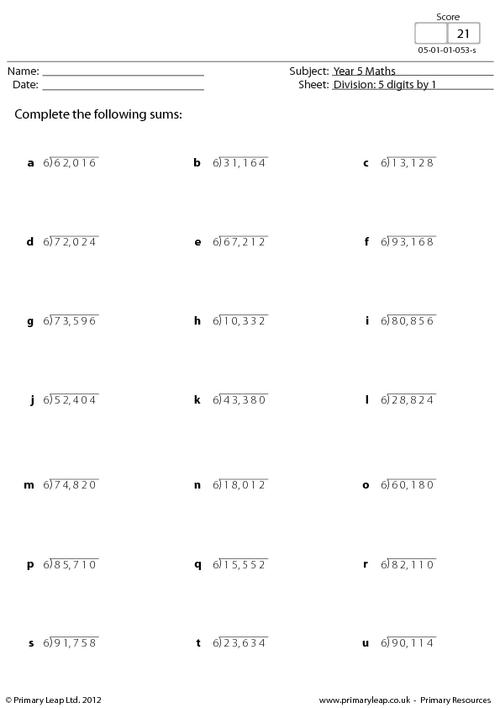### Division: 5 digits by 1 - set 1

Math: Division - 21 division questions dividing 5 digits by 1.

Age: 9 - 10
1
2
3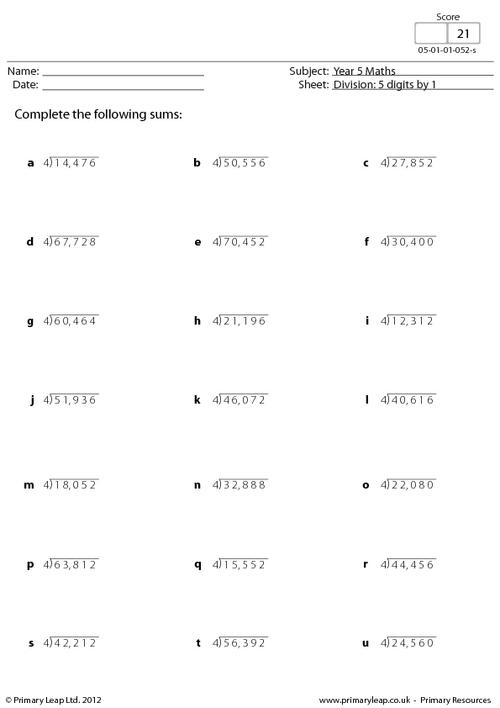### Related facts - Division by 12

Math: Division - These 8 division questions show how the same sum can be written in different ways/divide by 12.

Age: 9 - 10
1
2
3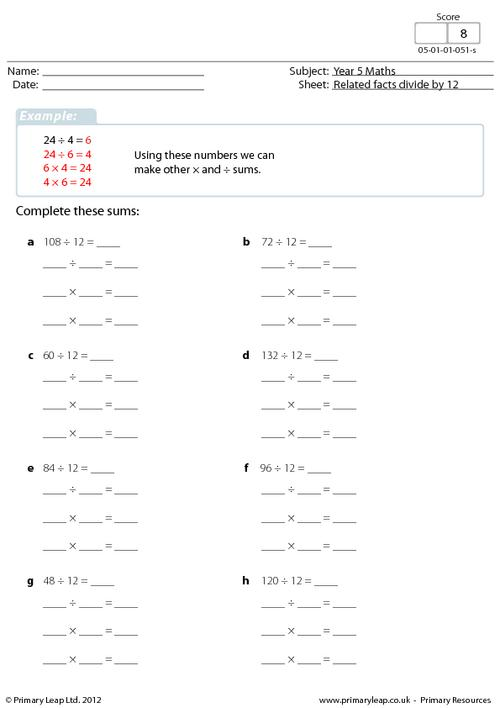### Related facts - Division by 11

Math: Division - These 8 division questions show how the same sum can be written in different ways/divide by 11.

Age: 9 - 10
1
2
3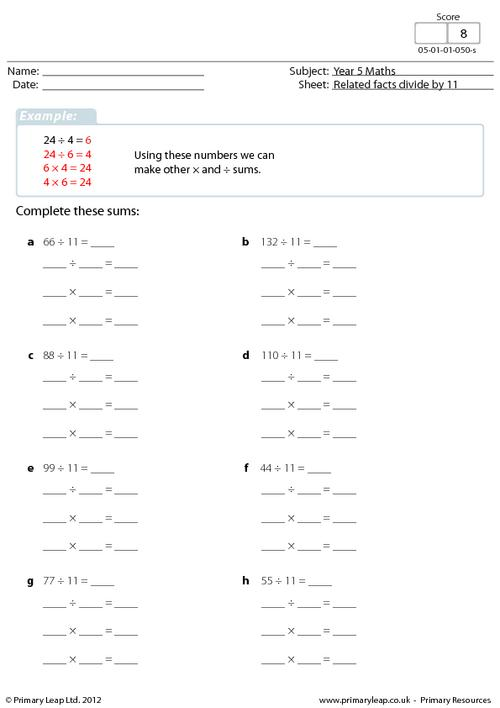### Related facts - Division by 10

Math: Division - These 8 division questions show how the same sum can be written in different ways/divide by 10.

Age: 9 - 10
1
2
3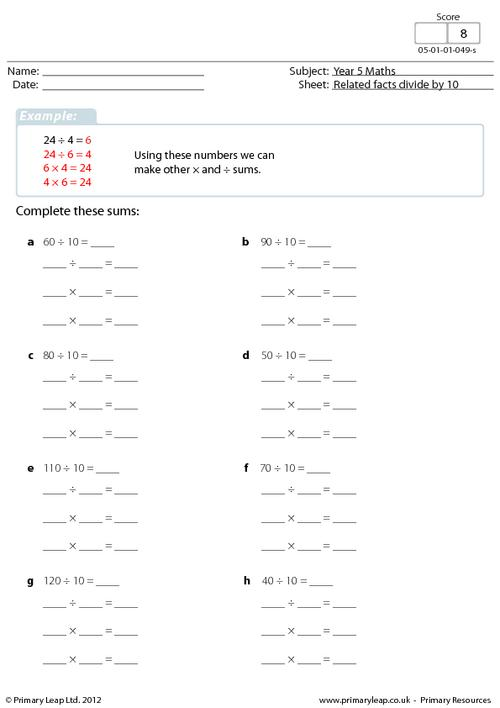### Related facts - Division by 9

Math: Division - These 8 division questions show how the same sum can be written in different ways/divide by 9.

Age: 9 - 10
1
2
3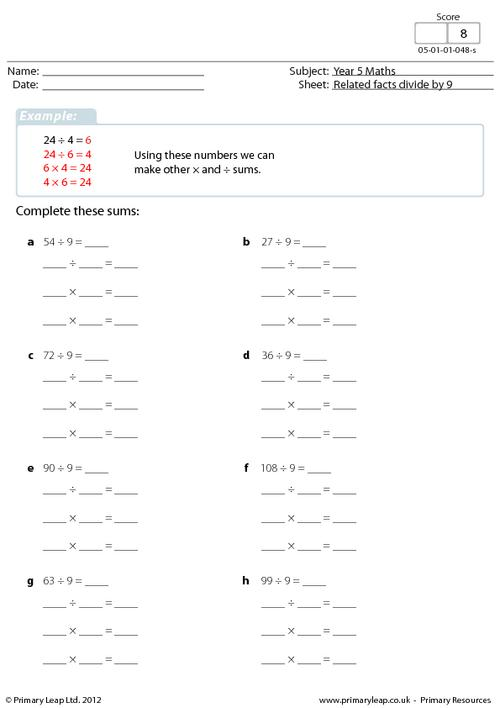### Related facts - Division by 8

Math: Division - These 8 division questions show how the same sum can be written in different ways/divide by 8.

Age: 9 - 10
1
2
3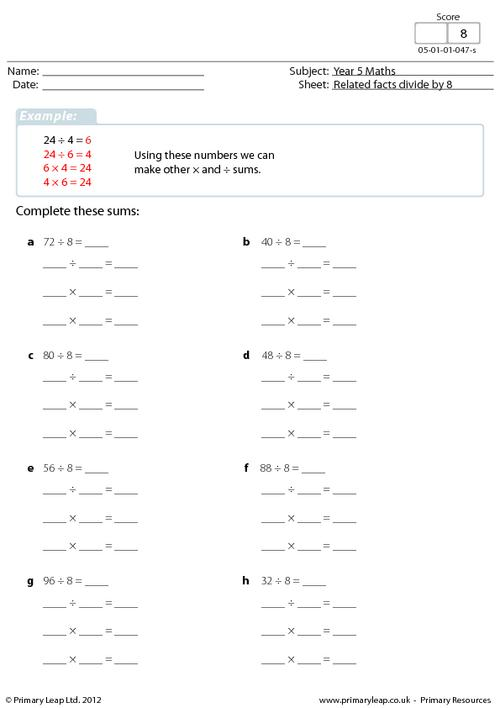### Related facts - Division by 7

Math: Division - These 8 division questions show how the same sum can be written in different ways/divide by 7.

Age: 9 - 10
1
2
3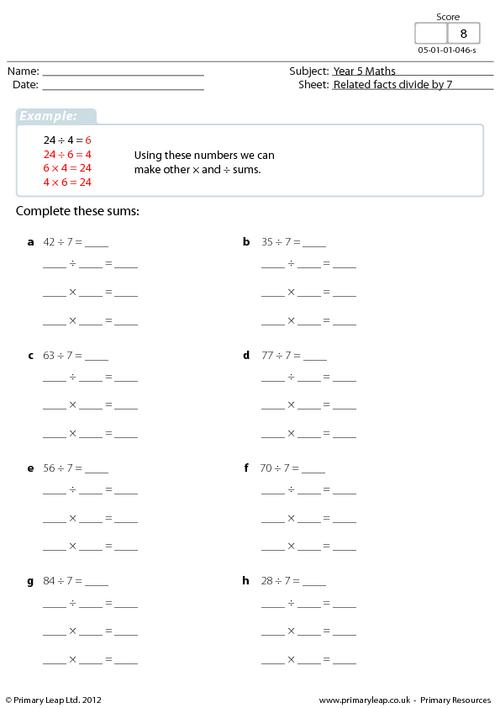### Related facts - Division by 6

Math: Division - These 8 division questions show how the same sum can be written in different ways/divide by 6.

Age: 9 - 10
1
2
3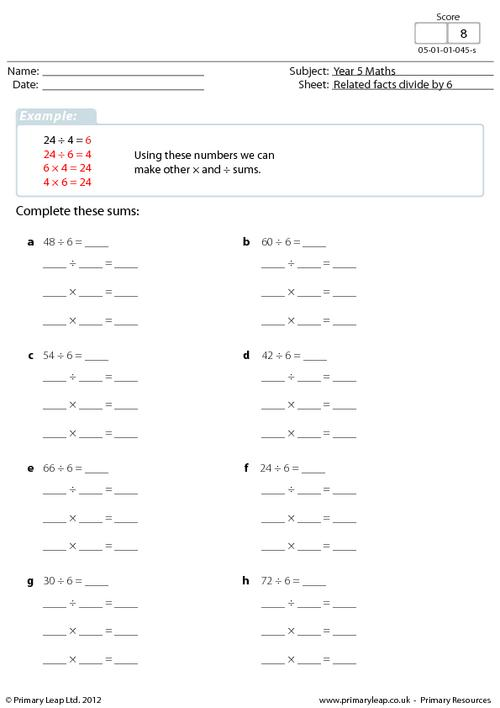### Related facts - Division by 5

Math: Division - These 8 division questions show how the same sum can be written in different ways/divide by 5.

Age: 9 - 10
1
2
3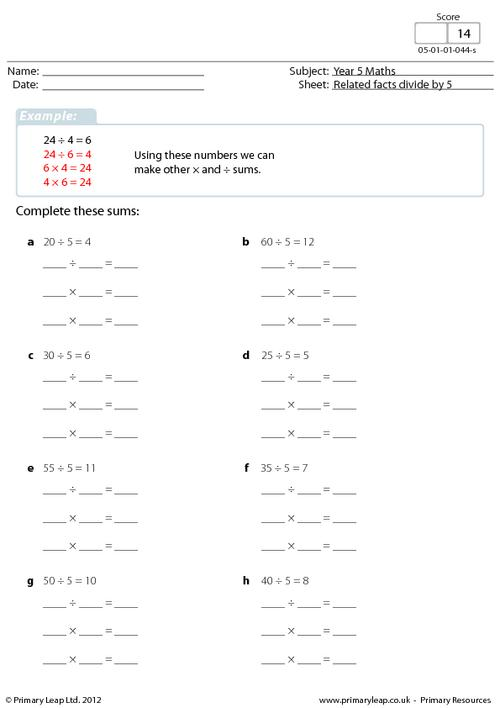### Short division with remainders 1

Math: Division - Solve the division sums with 2's and 5's/with remainders.

Age: 9 - 10
1
2
3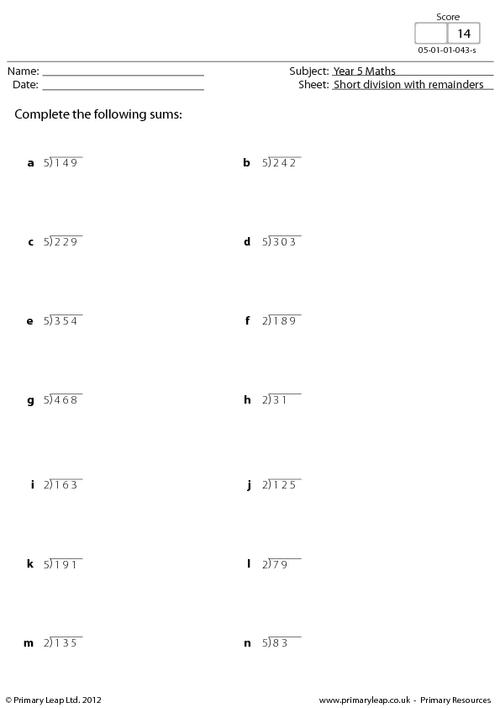### Short division 2

Math: Division - Solve the division facts with 2's and 5's/with remainders.

Age: 9 - 10
1
2
3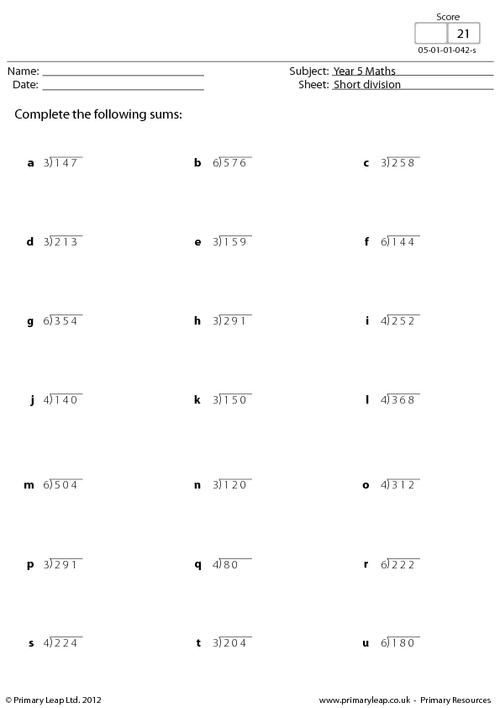### Short division

Math: Division - Solve the division facts with 7's, 8's and 9's/no remainders.

Age: 9 - 10
1
2
3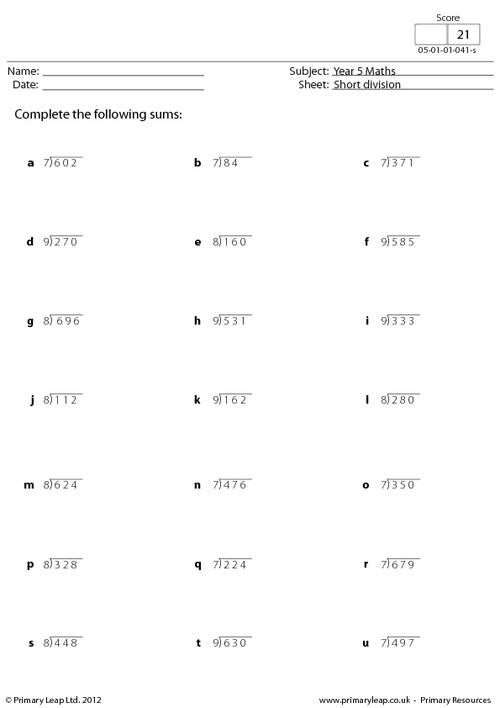### Triangular numbers info sheet

Math: Triangular numbers - Children can use this fact sheet about triangular numbers to help them with their sums.

Age: 9 - 10
1
2
3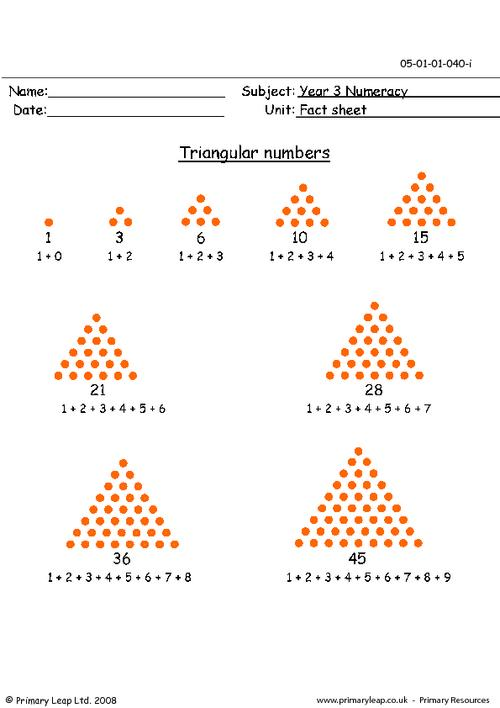### Square numbers info sheet

Math: Square numbers - Children can use this fact sheet to help them with their sums.

Age: 9 - 10
1
2
3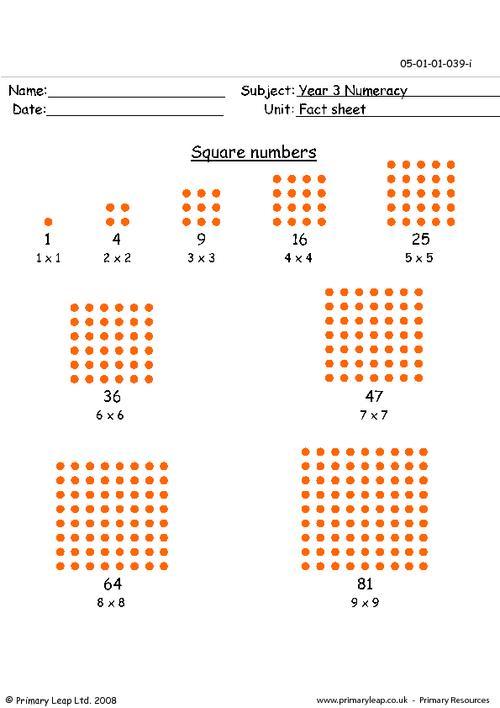### Money 1

Math: Money - Find the total cost of the given items in our hungry pet shop.

Age: 9 - 10
1
2
3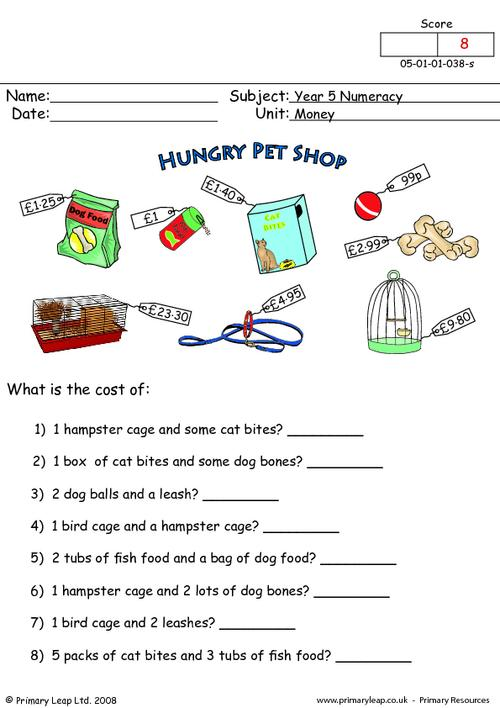### Division

Math: Division - Solve these division sums with and without remainders.

Age: 9 - 10
1
2
3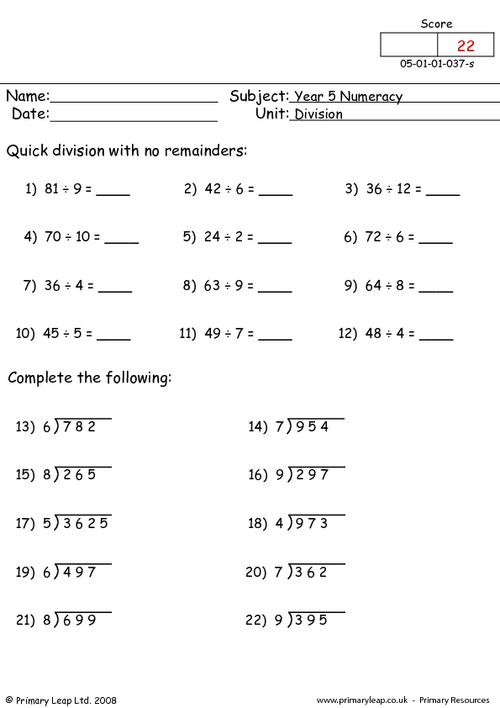### Long multiplication 2

Math: Long multiplication - Solve the 3 digit by 2 multiplication problems.

Age: 9 - 10
1
2
3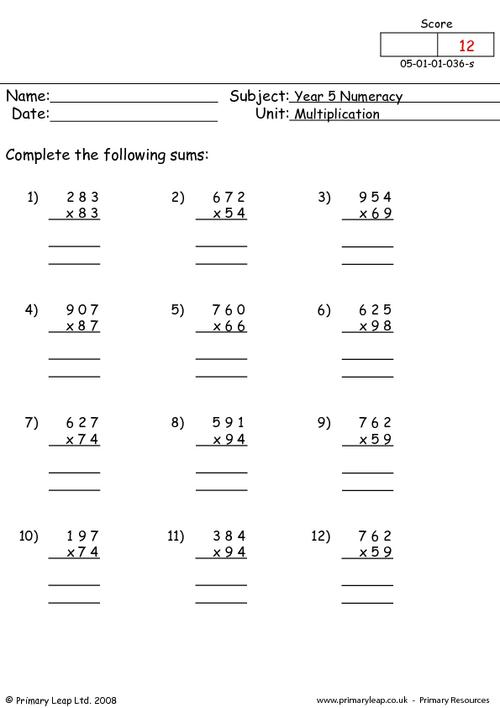### Long multiplication 1

Math: Long multiplication - Solve the 2 digit by 2 digit multiplication problems.

Age: 9 - 10
1
2
3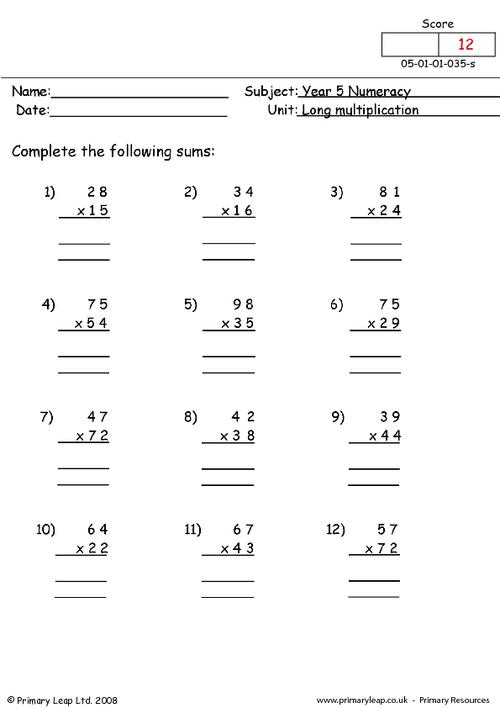### Multiplication squares

Math: Multiplication squares - Fill in the multiplication squares and see how well you know your times tables!

Age: 9 - 10
1
2
3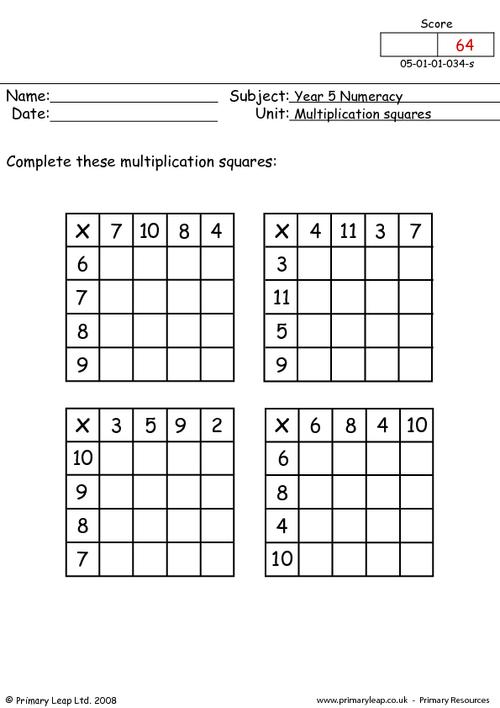### Multiplication

Math: Multiplication - Complete these multiplication sums and see how many you can get right!

Age: 9 - 10
1
2
3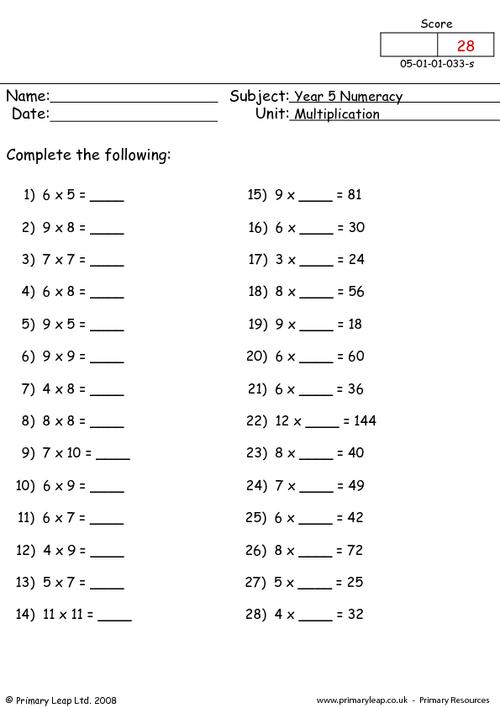### Subtraction

Math: Subtraction - Children must complete the various subtraction sums.

Age: 9 - 10
1
2
3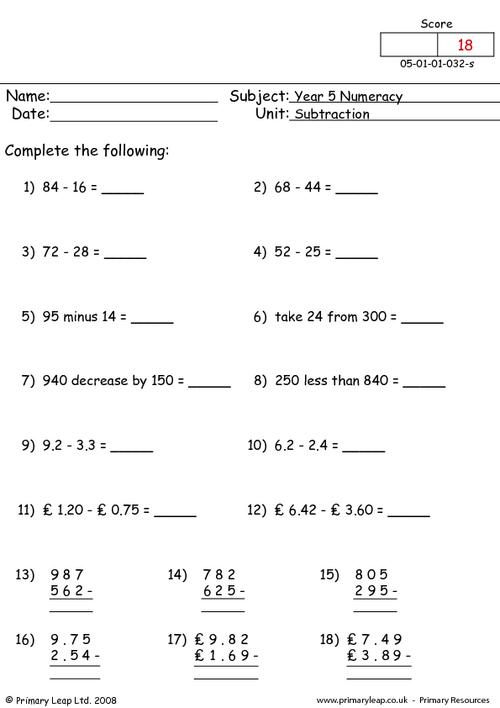Math: Addition problems - Complete the following written questions.

Age: 9 - 10
1
2
3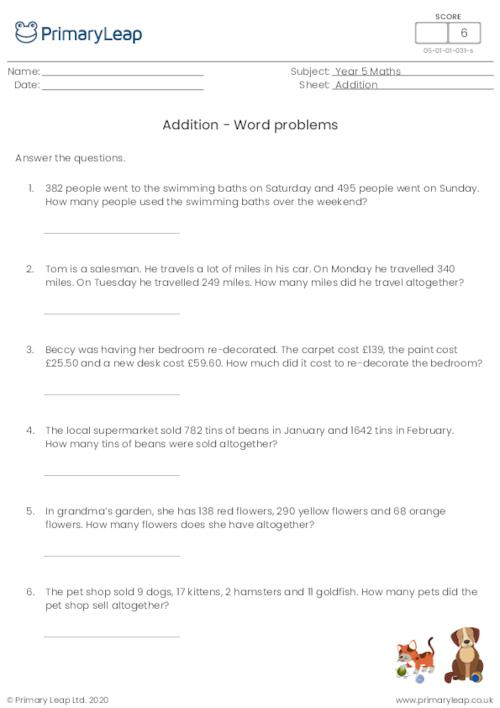Math: Addition - Fill in the missing numbers to complete the addition sums.

Age: 9 - 10
1
2
3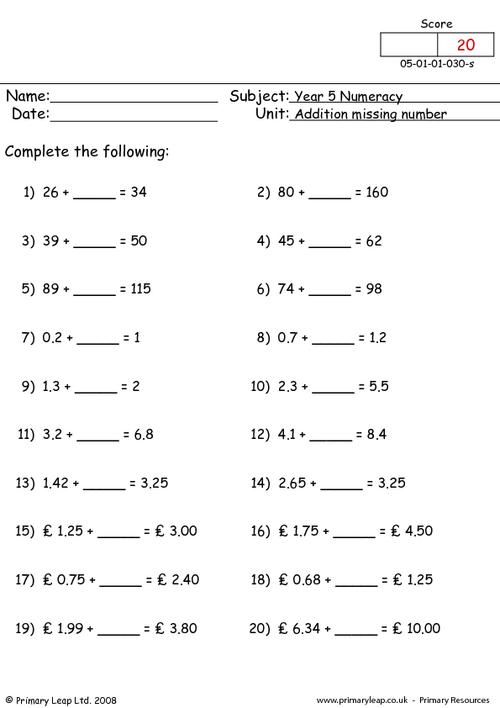Age: 9 - 10
1
2
3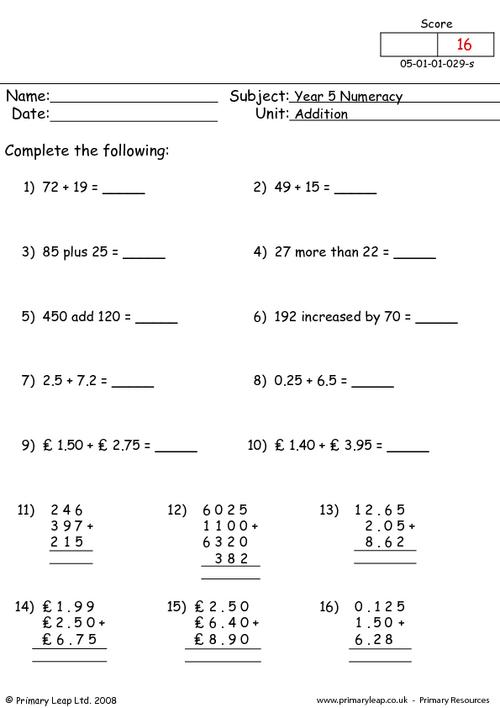### Adding to 100 and 1000

Math: Addition - Finding the pairs of numbers that equal 100 or 1000 and put them in the correct piggy bank.

Age: 9 - 10
1
2
3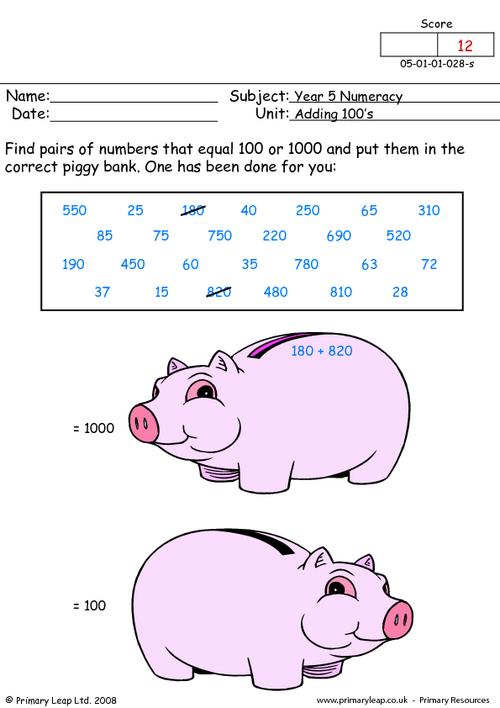### Percentages of quantities

Math: Percentages - Children must find the percentages of the given quantities.

Age: 9 - 10
1
2
3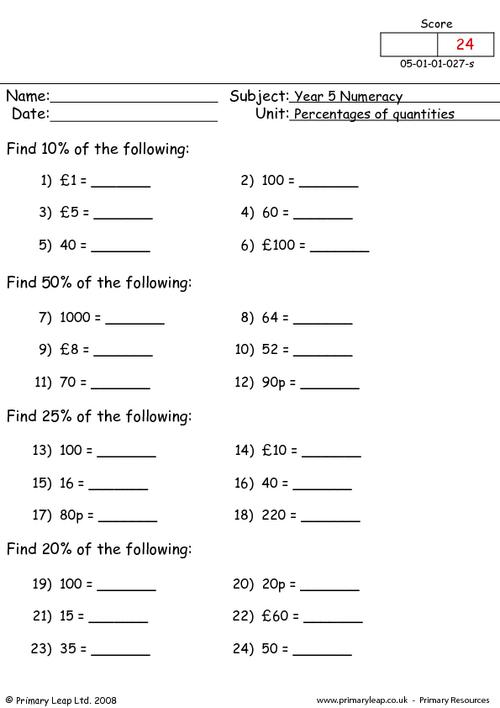### Fractions to percentages

Math: Fractions - Children must turn the fractions into percentages.

Age: 9 - 10
1
2
3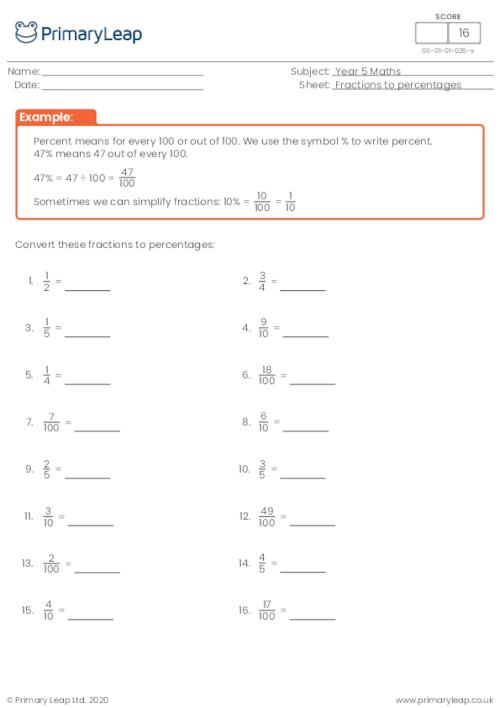of 2## Improve your child's mental and written calculation skills with our year 5 maths worksheets

In year 5, your child will continue to practise mental and written calculation methods and use all the operations to solve problems. Our fun and engaging maths worksheets will help your child to multiply fractions, learn more about decimals and percentages, round numbers up to 1,000,000, and much more.Learn

One of the best things about printable worksheets is that they make learning fun and easy. Explore our educational resources based on Year 5 Maths and keep your children learning through these fun and engaging exercises.Progress

Our Year 5 Maths printable worksheets are designed for progressive learning. Our engaging content enables a child to learn at their own speed and gain confidence quickly. When we help a child believe in themselves, we empower them for life.Improve

Printable worksheets are a great way to enhance creativity and improve a child’s knowledge. Help your child learn more about Year 5 Maths with this great selection of worksheets designed to help children succeed.